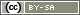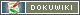This translation is older than the original page and might be outdated. See what has changed.

# Direct Feedback for Respondents

This chapter describes different application cases if respondents should get a direct feedback wether their answer is right or wrong after clicking on the next button. This instruction describes different use cases if respondents should receive feedback directly after clicking on “Continue” whether their answer was correct or incorrect. Such direct feedback is used in online training courses, for example.

There are several designs for feedbacks. This guide presents examples of some of these possibilities. However, the basic principle is always the same: By means of `value()`, the answer of the respondent(s) is read out of the data set and compared with the correct answer. The correct answer is coded directly in the PHP code or (for large projects with many questions) stored in the Database for Contents. Depending on the result of the comparison, PHP-Filter will then indicate that the answer is correct or not.

In case of a wrong answer, the correct answer can optionally be displayed and/or the respondents have to return to the previous page via “Back” and correct their answer.

This guide is devided in two parts. The first part explains how to check the correctness of the answer in different designs. The second part explains different variants of feedback.

Tip: If you want to output an overall result, e.g. the proportion of correct answers, then read the instructions Score Responses.

The matching between the given answer and the correct answer depends on two factors:

```* First of all, the answer format, here is particularly important whether one compares only one value (e.g. in a simple selection) or whether several values have to be compared (e.g. in a multiple selection or if the answer contains several individual answers).
* On the other hand, it is important for the implementation whether the feedback is only needed for a handful of questions or whether a longer catalogue of questions is involved. In the second case, it is useful to work with [[:en:create:array|arrays]] and ''[[:en:create:functions:looptopage]]'', so that you do not have to copy the PHP code many times.```

In each of the following examples, only one text “Your answer is correct” (text with the identifier “FB01”) or “Your answer is incorrect” (identifier “FB02”) is displayed as feedback. Further down in the instructions, it will be explained how to implement elaborate feedback.

The PHP code is placed on an additional page after the page with the actual question Ausnahme ist die Lösung mit `loopToPage()`. There, only two pages are needed for a whole series of questions, which are displayed several times: One page for the questions (a different question is shown in each repetition) and one page for the feedback.

### Single Choice

This basic comparison between an answer and the correct value is at the same time the basis for all further filters. The following PHP code checks whether the option with answer code 3 was selected in the selection question “AB01”:

```if (value('AB01') == 3) {
} else {
}```

### Multiple Choice

A multiple choice has one variable for each choice option. This can have the value 1 (not ticked) or 2 (ticked). In order to answer a multiple choice correctly, it must be checked for each choice option whether it has been answered correctly – i.e. whether it has been correctly selected or correctly left blank.

Take as an example a multiple choice AB02 with 4 answer options, of which the second and fourth must be ticked in order to answer correctly:

• (AB02_01) apple
• (AB02_02) aubergine
• (AB02_03) strawberry
• (AB02_04) potatoe

There now are two ways for checking. The first case connects 4 single answer checks via logical “and” (Linking Multiple Conditions). This is realtively simple but bulky

```if ((value('AB02_01') == 1) && (value('AB02_02') == 2) && (value('AB02_03') == 1) && (value('AB02_04') == 2)) {
} else {
}```

The same code can also be written a little more clearly, but that makes it only slightly more manageable:

```if (
(value('AB02_01') == 1) &&
(value('AB02_02') == 2) &&
(value('AB02_03') == 1) &&
(value('AB02_04') == 2)
) {
} else {
}```

It becomes more elegant if one defines the correct answer scheme as an array (`[1,2,1,2]`) and compares this list with the list of given answers as provided by `valueList()`. However, the function `array_values()` must still be used here, because `valueList()` also returns the variable identifiers that would interfere with the comparison.

```\$answer  = array_values(valueList('AB02'));
\$correct = [1,2,1,2];
} else {
}```

If you need a little more flexibility, you can also compare several variables with a FOR loop (see below).

### Comparison of Several Variables

If a page includes several questions, then this can also be solved with `valueList()` as shown above with the multiple selection. However, this is more time-consuming because the variable names may have to be specified individually.

As an example, let's take the two questions “AB01” and “AB02”, both of which must be answered correctly. So a 3 for the variable “AB01” and the values 1, 2, 1, 2 for the variables “AB02_01” to “AB02_04”.

```\$answer  = array_values(valueList(['AB01', 'AB02_01', 'AB02_02', 'AB02_03', 'AB02_04']));
\$correct = [3,1,2,1,2];
} else {
}```

An alternative solution is to use a FOR loop. The variable identifier and the correct answers are first defined as an array and then checked individually in the loop. WIf a comparison is wrong, the complete answer is wrong.

```\$solution = [
'AB01' => 3,
'AB02_01' => 1,
'AB02_02' => 2,
'AB02_03' => 1,
'AB02_04' => 2
];

\$allRight = true;
foreach (\$solution as \$varID => \$correct) {
if (value(\$varID) != \$correct) {
\$allRight = false;
}
}

if (\$allRight) {
} else {
}```

The reaction to a correct or incorrect answer can be designed quite differently. Essential building blocks are:

• The PHP Function `text()` to display a predefined text.
• The PHP Function `option('nextbutton', false)` to hide the Next button so that respondents have to go back to the previous page and correct their answer.
• The PHP Function `repeatPage()`, to show a message and return directly to the previous page.
• The PHP Functions `getItems()`, `getItemtext()` and `html()`, to redisplay the previous question and mark wrong/correct answer options.

### Show a Text

The simplest variant, namely to show a text “Your answer was correct”, has already been used several times above.

If you add a text to a rubric of your choice in the question catalogue and select the option “Formatted text” for the presentation, you can also design the text a little.

If you want to make the text a little more sophisticated, select the option “HTML code” at presentation and use HTML and CSS (format texts):

```<div style="border: 2px solid green; padding: 10px 16px; border-radius: 5px; background-color: #99FF99; text-align: center; Width: 50%; margin: 3em auto;">
</div>```

For further design you can also use images, see Images in the Questionnaire.

The integration of the text is done, as shown above, by means of `text()`. The following example assumes that the text for the positive feedback has the identifier “FB01” and the text for the negative feedback has the identifier “FB02”.

The PHP-Variable `\$allRight` would have been defined further up in the PHP code – something like in the example above. Of course, the IF filter can also contain a different condition (see above).

```if (\$allRight) {
text('FB01');
} else {
text('FB02');
}```

### Block Next Button

With the function `option('nextbutton', false)` you can also hide the back button if the answer is wrong.

```if (\$allRight) {
text('FB01');
} else {
text('FB02');
option('nextbutton', false);
}```

### Go Directly to the Previous Page

With the function `repeatPage()` you can also directly repeat the page with the question in case of a wrong answer.

```if (!\$allRight) {
repeatPage('FB02');
}```

The positive message would be obsolete in this case.

## Detailed Feedback

It is slightly more elaborate if you want to show the question and the wrong and right answers directly as feedback. The following PHP code first checks the answers to different questions “AB01” to “AB05” and then shows for each sub-question whether it was answered correctly or not.

```// Enter the questions and the correct answers here
'AB01' => 1,
'AB02' => 2,
'AB03' => 2,
'AB04' => 1,
'AB05' => 1
];

// Here follows the PHP code for the display
foreach (\$answers as \$question => \$correct) {
html('
<div class="question">
<div class="text">'.getItemtext(\$question, 'question').'</div>
');
if (\$antwort == \$correct) {
} else {
html('<div class="better">⚠️ '.getItemtext(\$question, \$correct).'</div>');
}
html('</div>');
}

// Here the layout
pageCSS('
div.question { margin-bottom: 3em; }
div.question div.text { font-weight: bold; margin-bottom: 0.5em; }
div.question div.correct { margin-bottom: 0.5em; color: green; }
div.question div.wrong { margin-bottom: 0.5em; text-decoration: line-through; }
div.question div.better { margin-bottom: 0.5em; color: green; font-weight: bold; }
');```

## Many Questions

If a relatively large number of questions are to be presented on individual pages, then the function `loopToPage()` is used. The list of questions including answers is defined in an array.

In a questionnaire are two pages needed. In the following example, the first page is given the pageidentifier “question”, the second page is given the page identifier “feedback”.

On the “question” page, the list of questions and correct answers is defined. In the following example for multiple choice questions “AB02” to “AB06”. By means of `registerVariable()` this array is also made available for page 2.

```\$questions = [
'AB02' => [1,2,1,2],
'AB03' => [2,2,1,1],
'AB04' => [2,1,1,2],
'AB05' => [1,2,2,1],
'AB06' => [1,1,1,2]
];
registerVariable(\$questions);

\$i = loopToPage('feedback', count(\$questions));

// show question
\$questionIDs = array_keys(\$question);
\$questionID = \$questionIDs[\$i];
question(\$question);```

The second page “feedback” then checks the answer and displays the feedback.

```\$i = loopIndex();
\$questionIDs = array_keys(\$questions);
\$questionID = \$questionIDs[\$i];

\$correct = \$questions[\$questionID];